Line Symmetry

A shape is said to have Line Symmetry (often called Reflection Symmetry or Mirror Symmetry) when it can folded in half so that both halves match. If a mirror is placed along the line of the fold (called the 'line of symmetry' or 'mirror line') then the shape will look the same as without the mirror.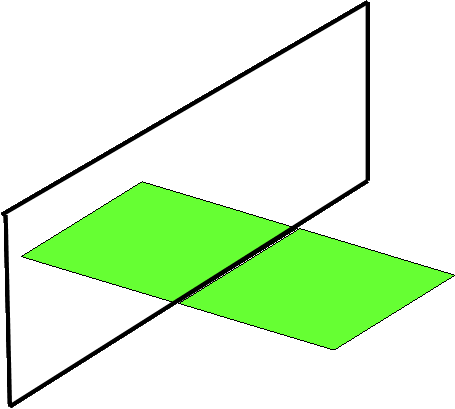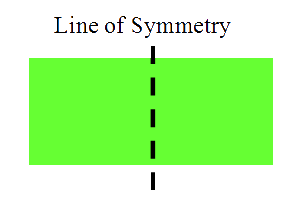Examples of lines of symmetry.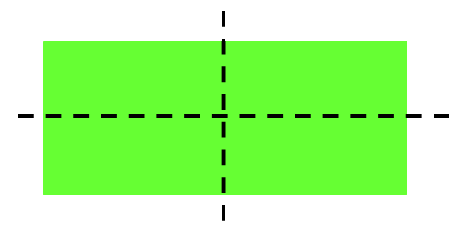Two lines of symmetry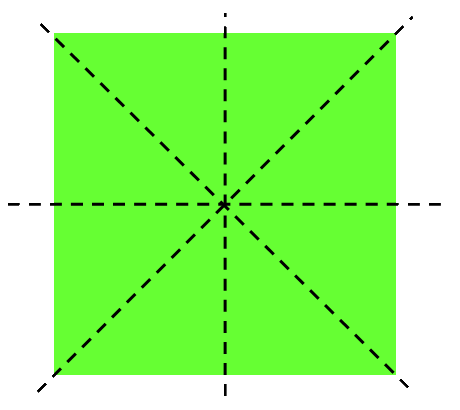Four lines of symmetry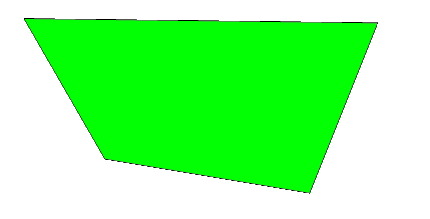No lines of symmetry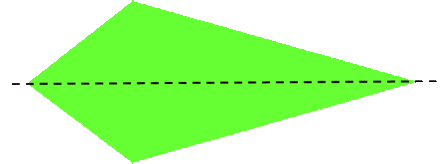One line of symmetry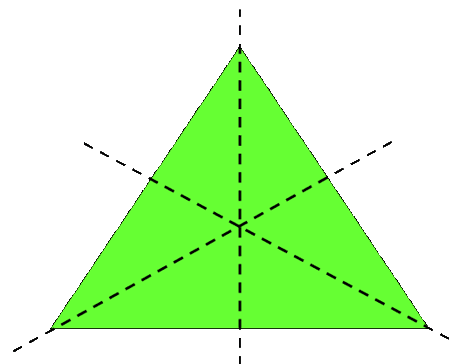Three lines of symmetry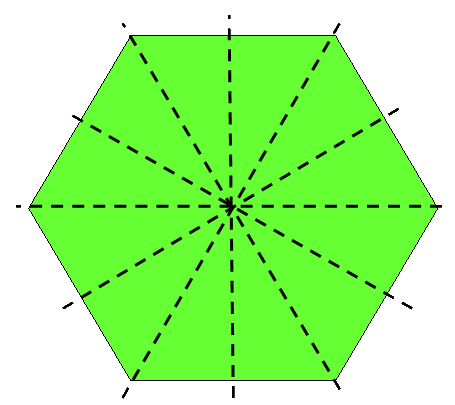Six lines of symmetry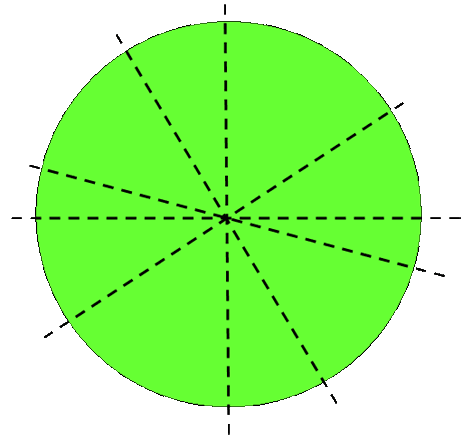Therefore this shape has an infinite number of lines of symmetry.

Practice working with Symmetry with these
worksheets from Primary Resources

Practice symmetry with this mission from BBC Bitesize

Play Full Screen version